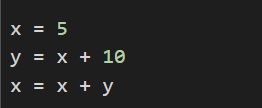# Python for Data Science: Introduction to DataFrames

One key aspect of Data Science is computation. Python, a programming language, is well suited for data science, specifically because of:

• The extensive collection of libraries that extend the functionality of the programming language for data science-related tasks,
• The relative ease of the syntax of the programming language (it does not look too cryptic!), and
• The wide-scale existing adoption of the Python language (there are millions of people who know Python and can help us out)

## Understanding Python Programs

Running Python programs requires a Python interpreter that will interpret your Python code and run it on a CPU. There are many Python interpreters that exist in the cloud -- such as Google Colab that we will use for online examples -- and you can install a Python interpreter on your own computer!

You will be using Python as a tool to help you preform data science on real-world data.

### Basic Python Data Types

The first and most important bit to know about Python is that there are just three basic data types that we will be using initially. A data type describes the type (or format) of the data and not the data itself. The three data types include:

1. Numbers, which include both integers (whole numbers like 4, 8, -3, and 0) and numbers with a decimal (floating point numbers like 3.14, 2.0001, and -42.1).

2. Strings, which includes all non-numeric data. Strings must be surrounded by quotes (ex: "Hello", "Ice Cream", "Sunshine").

3. DataFrames, which are tables that are similar to a spreadsheet containing rows and columns of data. Each DataFrame usually consists of an entire dataset or some subset of the full dataset.

Everything we initially work with will be one of the three types of data.

### Using Variables

Python allows us to store data inside of named variables, which allows us a way to store and interact with data. This is similar to basic mathematics where you might have:

• x = 5, the value of x is set to 5,
• y = x + 2, the value of y is set to the value of x (which is 5) plus 2 = 7, and
• z = x + y, the value of z is set to the sum of x (5) and y (7) = 12

Variables will store the value you assign to them until you assign them a new value. In the example above, x will always have the value of 5 until x is set again.

### DataFrames

In data science, variables that store DataFrames will contain the data from our datasets. To load in any data into a variable, we will always preform three steps:

1. We must first import the pandas library, giving us access to DataFrames. This is done via the import command and will always be the following line of code:
# Imports the pandas library to be used in our Python program:
import pandas as pd
1. We must then load the dataset, assigning a variable to become a DataFrame. Unless we are working with many datasets at the same time, it is common to call the variable storing the dataset df, short for DataFrame. The pd.read_csv(...) command loads the dataset from a provided URL. The code we will use is always the following:
# Loads the "GPA dataset" into the variable df using pd.read_csv:
df = pd.read_csv("https://waf.cs.illinois.edu/discovery/gpa.csv")
1. In any Python notebook environment, writing the name of the variable as the last line in a cell will always display the variable's contents to the screen. To verify we loaded our data correctly, we'll display the contents of the variable df that we just assigned to be a DataFrame in the previous step:
# Displays the contents of the variable df:
# (df contains the GPA dataset after the previous pd.read_csv(...) step)
df

## Running Python Programs

When you see Python code, the best way to understand what it's doing is to run it and nerd out with the code! Every page with code in Data Science Discovery will have a notebook just one click away:

The notebooks will be read-only notebooks that allow you to edit the code. You should create your own copies of the notebooks, code the notebooks, modify the code, etc. by using File -> Save a Copy in Drive. This will create your own private copy of the code that you can change, modify, and keep on your own private Google Drive!

In the labs and projects, you will use Python on your own computer and learn how to create files offline.

# Example Walk-Throughs with Worksheets

### Video 1: DataFrames

Follow along with the workseet to work through the problem:

# Practice Questions

Q1: Which of the following are all examples of data types in Python?
Q2: What best describes the code: "x = 4"?
Q3: Before loading a dataset, or using any other functionality in the panda's library, what Python code must run first?
Q4: What is the value of x after the following lines of code are run?